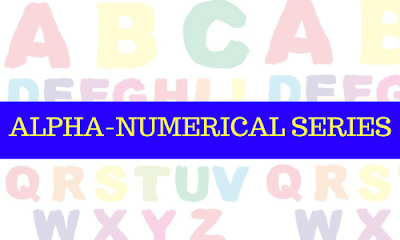New Students Offer - Use Code HELLO

# Alpha Numerical Series : Shortcut Tricks - Part 5In this article, we learn how to solve Alpha-numerical series easily by developing tricks

### Set 1:

A B C D E F G H I J K L M N O P Q R S T U V W X Y Z

### Question 1:

In above sequence, which letter or number or symbol will be mid way between 4th from left and 6th from right end?
TRICK: If both are in opposite direction (add)
=TO FROM
=4L + 6R = No such letter
TRICK: To find mid- way, answer is no such variable when
If both To and From are even or odd = No such letter

### Question 2:

In above sequence, which letter will be mid way between 9th from the left end and 8th from right end?
TRICK: If from either ends, one is even and another one is odd, then there is a possibility for mid way between them.
=TO FROM
=9L + 8R
=17R =26-17= 9/2 =4.5 =5(APPROX)
=9L+5L = 14L=N
(OR)
=8R + 5R =13R = N (Answer)

### Question 3:

Which letter will be midway between 8th from the left end and 10th letter from right end?
TRICK: To find mid- way, answer is no such variable when
If both To and From are even or odd = No such letter

### Question 4:

Which letter will be mid way between a 6th letter from left and the 9th letter from right end?
TRICK: If from either ends, one is even and another one is odd, then there is possibility for mid way between them.
=26-15=11/2=5.5=6 (Approx)
=TO FROM
=6L + 9R
=6L + 6L = 12L = L
(OR)
=9R + 6R = 15R = L(Answer)

### Tricks to Solve Alphanumeric Series Easily